# NCERT Solutions for Class 7 Maths Exercise 14.2## myCBSEguide App

CBSE, NCERT, JEE Main, NEET-UG, NDA, Exam Papers, Question Bank, NCERT Solutions, Exemplars, Revision Notes, Free Videos, MCQ Tests & more.

NCERT solutions for Maths Symmetry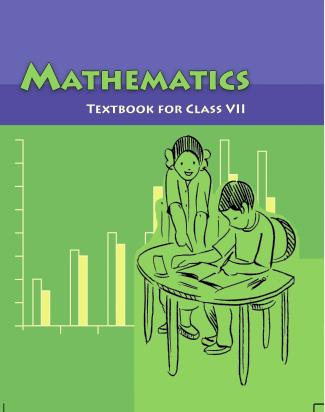## NCERT Solutions for Class 7 Maths Symmetry

Class –VII Mathematics (Ex. 14.2)

Question 1. Which of the following figures have rotational symmetry of order more than 1: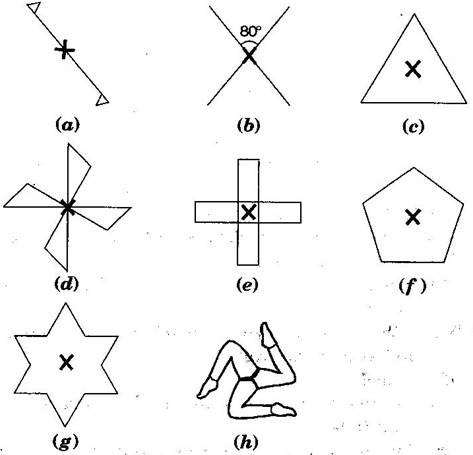Answer: Rotational symmetry of order more than 1 are {tex}\left( a \right),\left( b \right),\left( d \right),\left( e \right){/tex} and {tex}\left( f \right){/tex} because in these figures, a complete turn, more than 1 number of times, an object looks exactly the same.

Question 2. Give the order the rotational symmetry for each figure: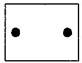S.No. Problem figures Rotational figures Order of rotational symmetry (a)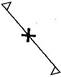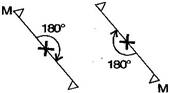2 (b)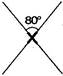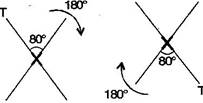2 (c)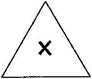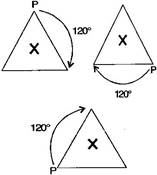3 (d)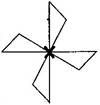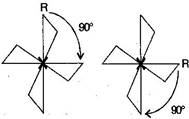4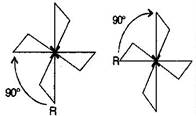(e)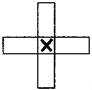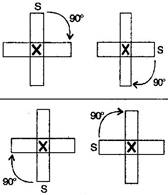4 (f)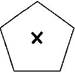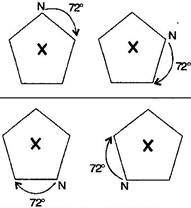5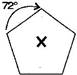(g)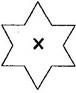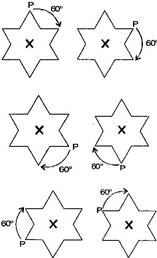6 (h)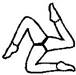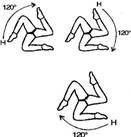3

## NCERT Solutions for Class 7 Maths Exercise 14.2

NCERT Solutions Class 7 Maths PDF (Download) Free from myCBSEguide app and myCBSEguide website. Ncert solution class 7 Maths includes text book solutions from Class 7 Maths Book . NCERT Solutions for CBSE Class 7 Maths have total 15 chapters. 7 Maths NCERT Solutions in PDF for free Download on our website. Ncert Maths class 7 solutions PDF and Maths ncert class 7 PDF solutions with latest modifications and as per the latest CBSE syllabus are only available in myCBSEguide.

## CBSE app for Students

To download NCERT Solutions for Class 7 Maths, Social Science Computer Science, Home Science, Hindi English, Maths Science do check myCBSEguide app or website. myCBSEguide provides sample papers with solution, test papers for chapter-wise practice, NCERT solutions, NCERT Exemplar solutions, quick revision notes for ready reference, CBSE guess papers and CBSE important question papers. Sample Paper all are made available through the best app for CBSE students and myCBSEguide website.### 4 thoughts on “NCERT Solutions for Class 7 Maths Exercise 14.2”

1. The figure given in the ?1st ques. Is the 2nd ques. Figure

2. Thank you

3. Thanks for helping everyone’s

4. Good job thank you you helped everyone ?????